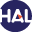Home page > 08. Volume 5 N°1 (2008) > Convergence analysis of an MPFA method

# Convergence analysis of an MPFA method

Sunday 6 December 2009,

Full Title:

Convergence analysis of an MPFA method for flow problems in anisotropic heterogeneous porous media

Abstract:

Our purpose in this paper is to present the theoretical analysis of a Multi-Point Flux Approximation method (MPFA method).

We start with the derivation of the discrete problem, and then we give a result of existence and uniqueness of a solution for that problem. As in finite element theory, Lagrange interpolation is used to define three classes of continuous and locally polynomial approximate solutions. For analyzing the convergence of these different classes of solutions, the notions of weak and weak-star MPFA approximate solutions are introduced.

Their theoretical properties, namely stability and error estimates (in discrete energy norms, L2 norm and L(infinity) norm), are investigated. These properties play a key role in the analysis (in terms of error estimates for diverse norms) of different classes of continuous and locally polynomial approximate solutions mentioned above.Voir sur HAL : https://hal.archives-ouvertes.fr/hal-01120075